# Quiz 12: Managerial Decisions for Firms With Market Power

The following is the information given about E. D. Star: a ) Table 1.1 shows the spreadsheet with the given inputs and cost structure: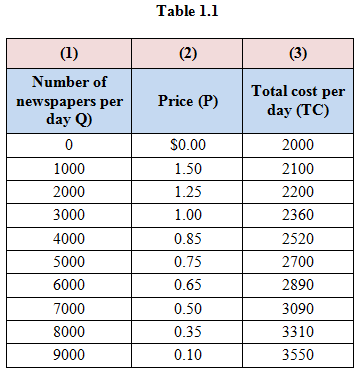b ) Write the formulae as follows: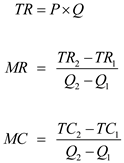Represent the values of Total Revenue ( TR ), Marginal Revenue ( MR ) and Marginal Cost ( MC ) of firm as follows: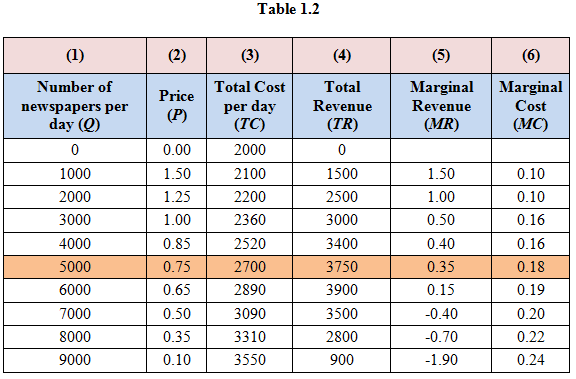Represent the table of the formulae excel sheet as follows: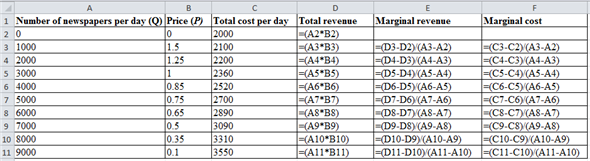The profit is maximized when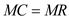. In the given case, MR and MC do not equal each other at any point, the nearest they can reach is when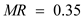and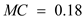. After this point, MC starts exceeding MR. This maximization of output happens when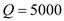and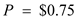. c) The firm is making highest revenue when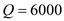. This is because monopolist does not work like competitive sellers. They charge differential prices to attain maximum profits. ( d ) Table 1.3 shows the total profit and profit margin: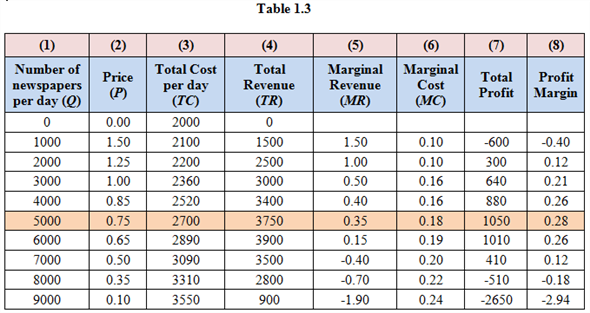Used Formulas to calculate values of table 1.2 as follows:Calculation part: For example calculate total profit when quantity is 2000 and price is \$1.25 as follows:Similarly, remaining total profit values can be calculated. For example calculate profit margin when quantity is 2000 and price is \$1.25 as follows:Similarly, remaining profit margin values can be calculated. Therefore, the profit and profit margin are maximized at quantity 5000. ( e ) The total fixed is \$2,000 for the firm. This is because when output is zero, total cost is \$2,000. Represent the formulae excel sheet as follows;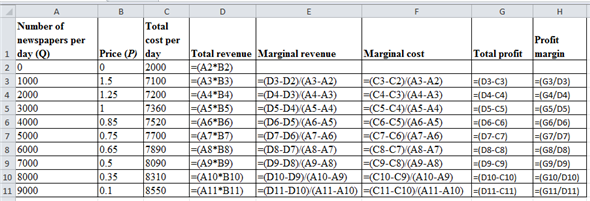Represent the table of the profit and profit margin when total fixed cost increases to 5,000 as follows: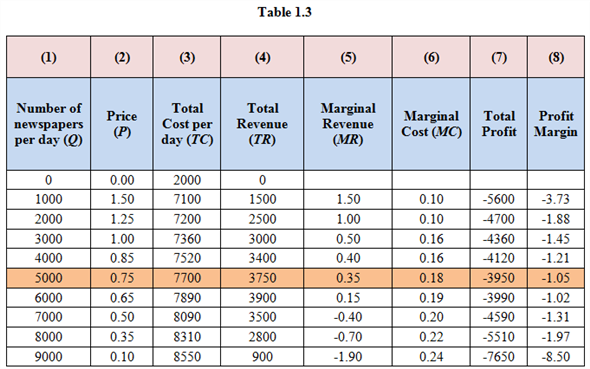Though the marginal cost and revenue intersect at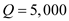, the profit margin is negative for all quantities. - In short run, price is greater than average variable cost, so the firm should not shut down. Otherwise firm will incur losses equal to fixed cost, \$5,000. - The fixed cost will spread over quantities in the long run, the profits will increase.